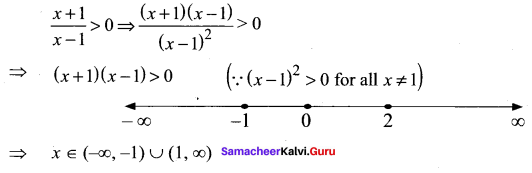## Tamilnadu Samacheer Kalvi 11th Maths Solutions Chapter 2 Basic Algebra Ex 2.5

Question 1.
Solve 2x2 + x – 15 ≤ 0.
Solution:
To find the solution of the inequality
ax2 + bx + c ≥ 0 or ax2 + bx +c ≤ 0 (for a > 0)
First we have to solve the quadratic equation ax2 + bx + c = 0
Let the roots be a and P (where a < P)
So for the inequality ax2 + bx + c ≥ 0 the roots lie outside α and β
(i.e.,) x ≤ α and x ≥ β
So for the inequality ax2 + bx + c ≤ 0. The roots lie between α and β
(i.e.,) x > α and x < β (i.e.) a ≤ x ≤ β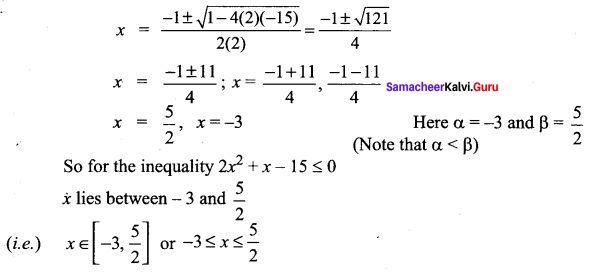Question 2.
Solve -x2 + 3x – 2 ≥ 0
Solution:
-x2 + 3x – 2 ≥ 0 ⇒ x2 – 3x + 2 ≤ 0
(x – 1) (x – 2) ≤ 0
[(x – 1) (x – 2) = 0 ⇒ x = 1 or 2. Here α = 1 and β = 2. Note that α < β]
So for the inequality (x – 1) (x – 2) ≤ 2
x lies between 1 and 2
(i.e.) x ≥ 1 and x ≤ 2 or x ∈ [1, 2] or 1 ≤ x ≤ 2

### Samacheer Kalvi 11th Maths Solutions Chapter 2 Basic Algebra Ex 2.5 Additional Questions

Question 1.
Solve for x.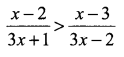Solution: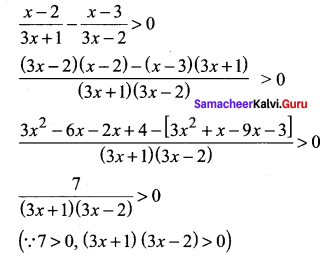Select the intervals in which (3x +1) (3x – 2) is positive
(3x + 1) > 0 and (3x – 2) > 0 or
3x +1 < 0 and 3x – 2 < 0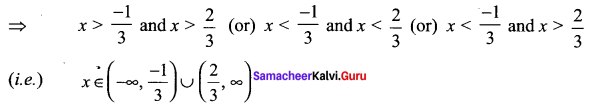Question 2.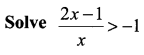Solution: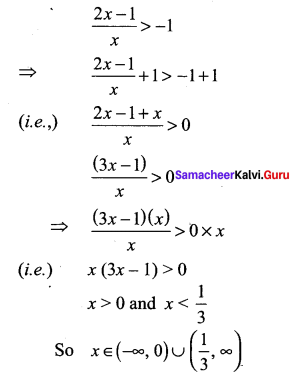Question 3.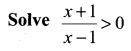Solution: